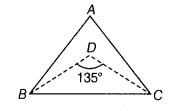# If the bisectors of the base angles of a triangle enclose an angle of 135°

If the bisectors of the base angles of a triangle enclose an angle of 135°,then prove that the triangle is a right angled triangle.

.Given In ∆ABC, bisectors of ∠B and ∠C form a triangle of angle 135°,Hence, ∆ABC is a right angled triangle